# ISEE Middle Level Math : How to find the square root

## Example Questions

← Previous 1 3 4 5 6 7 8 9 30 31

### Example Question #1 : How To Find The Square Root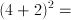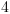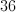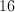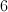Explanation:

Follow PEMDAS for order of operations.  First, we add the numbers inside the parentheses.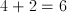Then we take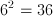.

### Example Question #2 : How To Find The Square Root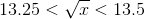Which of the following is a possible value for?

None of the other answers are correct.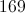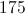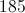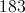None of the other answers are correct.

Explanation:We can find more information on the value ofby squaring all of our terms to remove the square root.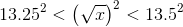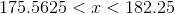Now we have a range of values for, however, none of these choices fall in this range.

### Example Question #3 : How To Find The Square Root

Evaluate: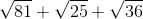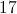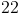Explanation:

Find the individual square roots and add them.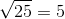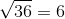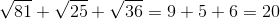### Example Question #2 : How To Find The Square Root

What is the product of the square root of twice eighteen and four less than ten?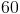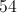Explanation: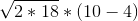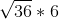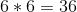### Example Question #1 : Squares / Square Roots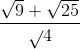=Explanation:

First find the square root for each number. Then solve accordingly.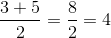### Example Question #3 : How To Find The Square Root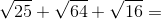Explanation:

First, find the square root of each number, then add.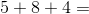### Example Question #7 : How To Find The Square Root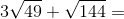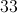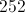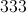Explanation:

First, find the square roots of the numbers: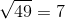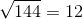Then, solve the equation using order of operations: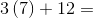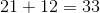The answer is.

### Example Question #8 : How To Find The Square Root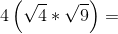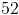Explanation:

First, find the square root for each: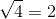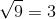Then insert these into the equation and solve: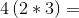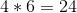The answer is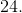### Example Question #5 : Isee Middle Level (Grades 7 8) Mathematics Achievement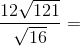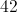Explanation:

First, find the square root for each number: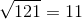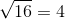Then, insert these square roots into the equation and solve accordingly: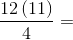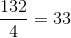### Example Question #4 : How To Find The Square Root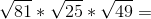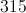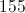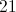Explanation:

First, find the square root of each number: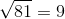Solve the equation accordingly: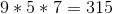← Previous 1 3 4 5 6 7 8 9 30 31